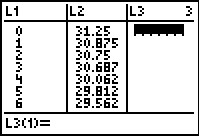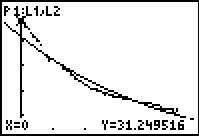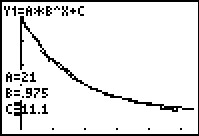••• ##### Device
• TI-83 Plus Family
• TI-84 Plus
• TI-84 Plus Silver Edition
•TI-84 Plus C Silver Edition
•TI-84 Plus CE
• ##### Software

TI Connect™
TI Connect™ CE

# Precalculus: How Cool It Is!

by Texas Instruments - Bell Ringer Lesson#### Overview

In this activity, students will use a temperature probe to generate a cooling curve and develop an appropriate regression equation to model collected data.

#### Key Steps

•In this activity, students engage in an exploration of the cooling of water. Data is imported into the list editor directly via the use of a temperature probe. They will use a variety of the graphing calculator’s features to obtain a best fit equation for the generated data.

•Visual inspection combined with exponential regression and algebraic manipulation of data results in modeling equations. Students evaluate their results both visually and by inspection of correlation coefficient values.

•Students further explore the exponential equation via the use of the Transformation Graphing application to visually obtain an appropriate model. For the second problem, students can use either the given data or the temperature probe to explore heating water.